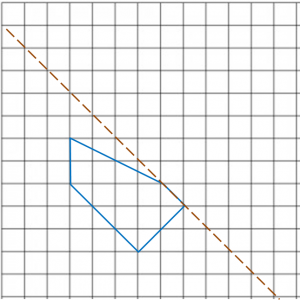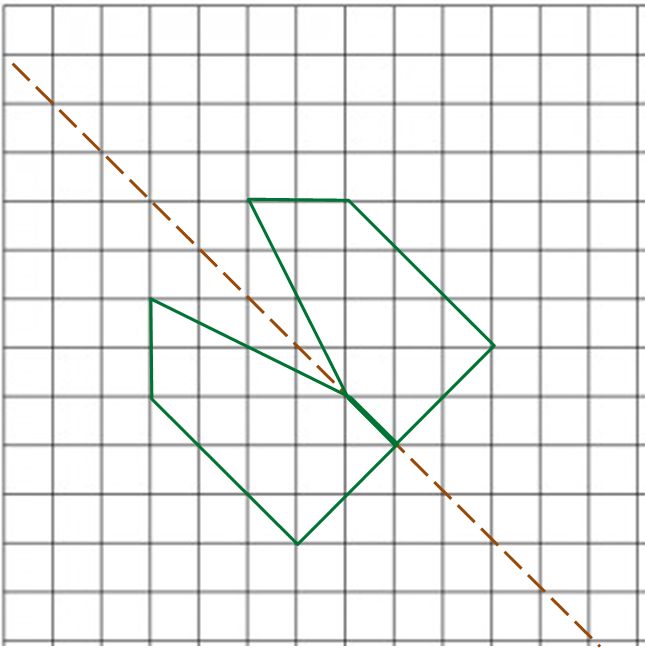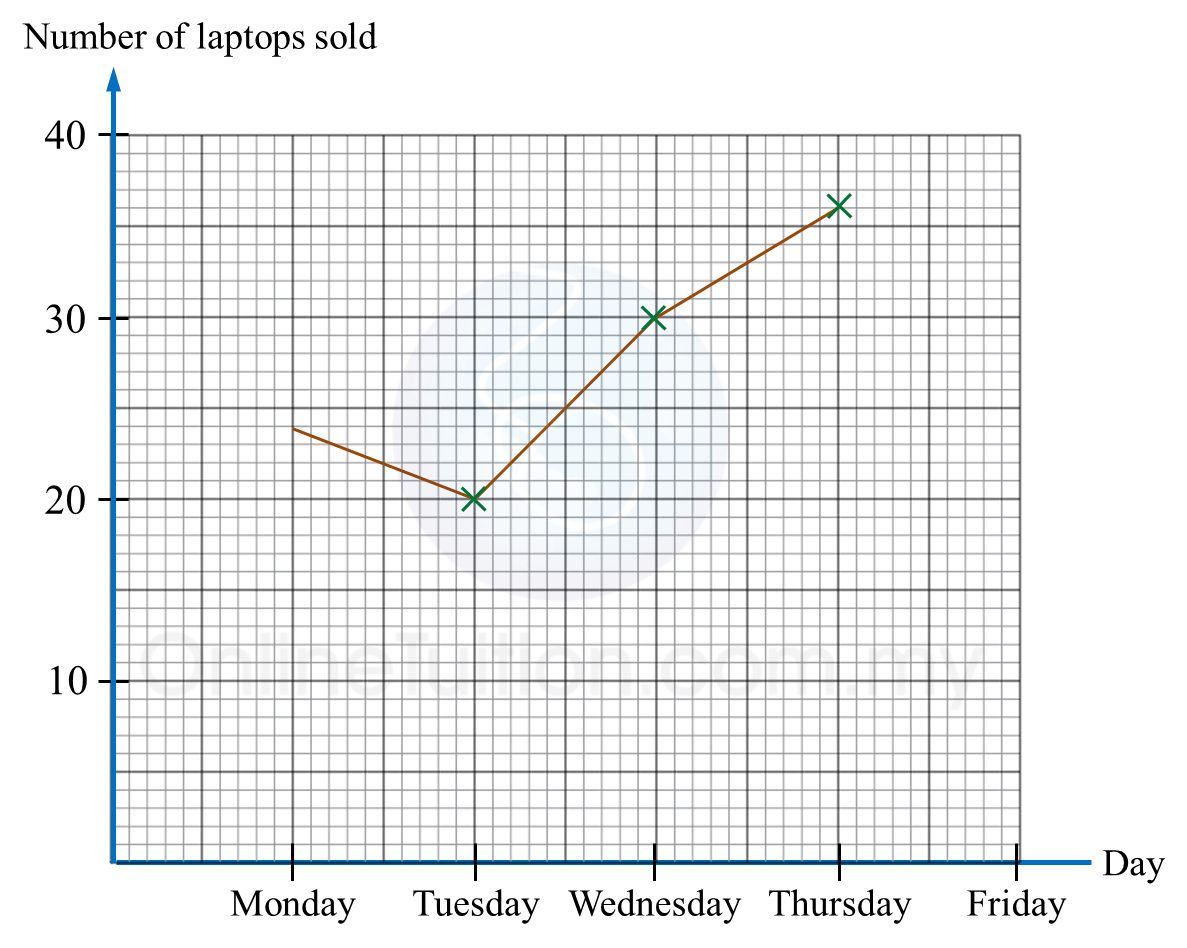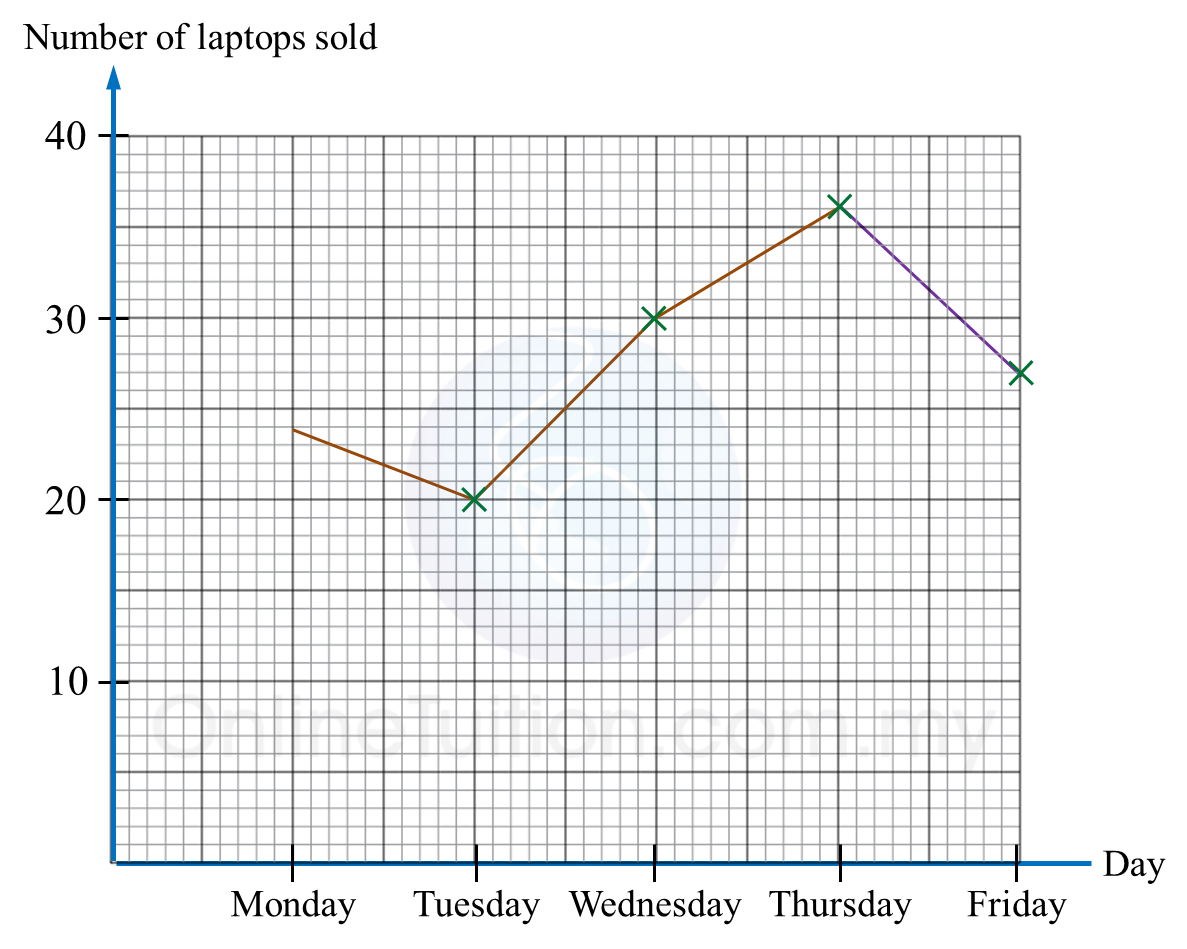# PT3 Mathematics 2016, Question 1

Question 1 (a):
Fill in the blanks.
(i) 3.5 cm = ______ mm
(ii) 6 500 ml = ______ l
(iii) 90 minutes = ______ hour

Solution:
(i)

(ii)

(iii)

Question 1 (b):
(i) Using a ruler, draw an axis of symmetry on diagram 1.1.(ii) Diagram 1.2 shows an incomplete polygon drawn on a square grid. The dotted line is the axis of symmetry.
Complete the polygon in the answer space.Solution:
(i)(ii)Question 1 (c):
Diagram 1.3 is an incomplete line graph showing the number of laptops sold in five days. The sales on Friday was ¾ of the total sales on Thursday.(a) Complete the line graph in the Diagram 1.3.
(b) Find the difference in the number of laptops sold between Thursday and Monday.

Solution:
(i)(ii)
Difference in the number of laptops sold
= 36 – 24
= 12